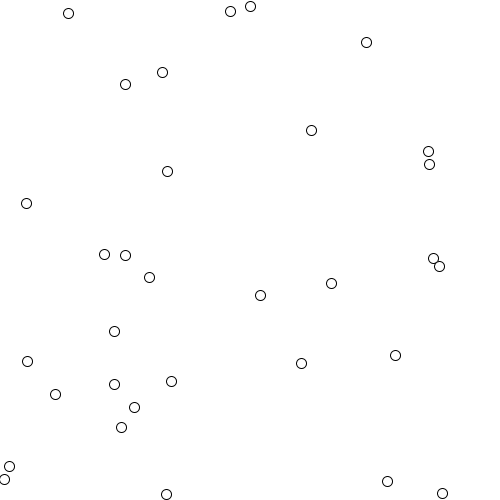# Common Fate

processing, motion, chance, class, repeat, Law of Common Fate
Visualizes the "Law of Common Fate". Elements with the same directional motion and/or same speed are seen as a group.``````// a simple particle system to visualize
// the law of common fate
// https://en.wikipedia.org/wiki/Gestalt_psychology#Gestalt_laws_of_grouping
// Law of Common Fate—The law of common fate states that objects are perceived as lines that move along the smoothest path. Experiments using the visual sensory modality found that movement of elements of an object produce paths that individuals perceive that the objects are on. We perceive elements of objects to have trends of motion, which indicate the path that the object is on. The law of continuity implies the grouping together of objects that have the same trend of motion and are therefore on the same path. For example, if there are an array of dots and half the dots are moving upward while the other half are moving downward, we would perceive the upward moving dots and the downward moving dots as two distinct units.
//
ParticleSystem sys; // the particle system

/**
* the setup runs once
*/
void setup() {
size(500, 500); // setup the canvas
sys = new ParticleSystem(); // create Particle System
// add to particles that share the same velocity vector
sys.add(new Particle(new PVector(width/2 -20, 10), new PVector(0, 1), color(255)));
sys.add(new Particle(new PVector(width/2, 5), new PVector(0, 1.2), color(255)));
sys.init(30); // init it with some more particles
}

/**
* the draw runs all the time
*/
void draw() {
background(255); // clear the background
sys.run(); // run the system
// saveFrame("seq/out-####.png");
// if(frameCount == 250) exit();
}
/**
* the particle system cass
*/
class ParticleSystem {
ArrayList <Particle> particles; // holds all the particles
/**
* ParticleSystem constructor
*/
ParticleSystem() {
this.particles = new ArrayList<Particle>(); // init the list
}
/**
* initialize the system with a set number of particles
* @param  {int} int num The number of particles to create
*/
void init(int num){
// for the given number
for(int i = 0; i < num; i++){
// the initial position
PVector position = new PVector(random(width), random(height));
// the velocity of that particle
PVector velocity = new PVector(random(-1,1),random(-1,1));
// create it
Particle p = new Particle(position, velocity, color(255));
// add it to the list
}
}

/**
* add a Particle to the list of particles
* @param {Particle} Particle p the Particle to add
*/
void add(Particle p) {
}
/**
* run the whole system
* this function also removes particles that are no longer on the canvas
* and ends the program if there are no more particles
*/
void run() {
// loop the list of particles and update their position
// and display them
for (int i = 0; i < this.particles.size(); i++) {
this.particles.get(i).update();
this.particles.get(i).draw();
}
// check if the particles are out of the canvas
// if so remove them from the list
for(int j = this.particles.size() -1; j >=0;j--){
if(this.particles.get(j).pos.x < 0 || this.particles.get(j).pos.x > width || this.particles.get(j).pos.y < 0 || this.particles.get(j).pos.y > height){
this.particles.remove(j); // he's dead jim
}
}
// end the program if the particles are all gone
if(this.particles.size()<1){
exit();
}
}
};

/**
* a simple particle class
*/
class Particle {
PVector pos; // the position
PVector vel; // the velocity
color col; // the color
/**
* Particle constructor
* @param {PVector} PVector p the initial position
* @param {PVector} PVector v the velocity
* @param {color} color   c the color of that particle
*/
Particle(PVector p, PVector v, color c) {
this.pos = p; // assign it to the local variables
this.vel = v; // assign it to the local variables
this.col = c; // assign it to the local variables
}
/**
* update the position of the particle
*/
void update() {